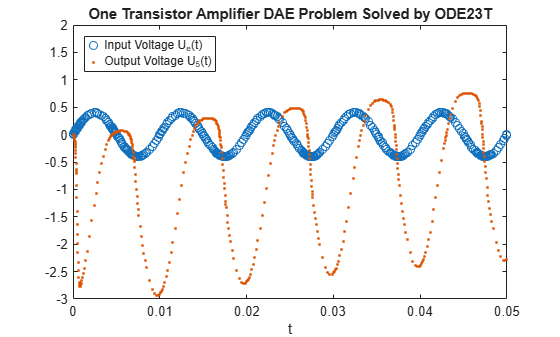# Solve Stiff Transistor Differential Algebraic Equation

This example shows how to use `ode23t` to solve a stiff differential algebraic equation (DAE) that describes an electrical circuit . The one-transistor amplifier problem coded in the example file amp1dae.m can be rewritten in semi-explicit form, but this example solves it in its original form $M{u}^{\prime }=\varphi \left(u\right)$. The problem includes a constant, singular mass matrix $\mathit{M}$.

The transistor amplifier circuit contains six resistors, three capacitors, and a transistor.• The initial voltage signal is ${U}_{e}\left(t\right)=0.4\mathrm{sin}\left(200\pi t\right)$.

• The operating voltage is ${\mathit{U}}_{\mathit{b}}=6$.

• The voltages at the nodes are given by ${\mathit{U}}_{\mathit{i}}\left(\mathit{t}\right)\text{\hspace{0.17em}\hspace{0.17em}}\left(\mathit{i}=1,2,3,4,5\right)$.

• The values of the resistors ${\mathit{R}}_{\mathit{i}}\text{\hspace{0.17em}\hspace{0.17em}}\left(\mathit{i}=1,2,3,4,5,6\right)$ are constant, and the current through each resistor satisfies $\mathit{I}=\mathit{U}/\mathit{R}$.

• The values of the capacitors ${\mathit{C}}_{\mathit{i}}\text{\hspace{0.17em}\hspace{0.17em}}\left(\mathit{i}=1,2,3\right)$ are constant, and the current through each capacitor satisfies $\mathit{I}=\mathit{C}\cdot \mathrm{dU}/\mathrm{dt}$.

The goal is to solve for the output voltage through node 5, ${\mathit{U}}_{5}\left(\mathit{t}\right)$.

To solve this equation in MATLAB®, you need to code the equations, code a mass matrix, and set the initial conditions and interval of integration before calling the solver `ode23t`. You can either include the required functions as local functions at the end of a file (as done here), or save them as separate, named files in a directory on the MATLAB path.

### Code Mass Matrix

Using Kirchoff's law to equalize the current through each node (1 through 5), you can obtain a system of five equations describing the circuit:

`$\begin{array}{l}\mathrm{node}\text{\hspace{0.17em}}1:\text{\hspace{0.17em}\hspace{0.17em}}\frac{{\mathit{U}}_{\mathit{e}}\left(\mathit{t}\right)}{{\mathit{R}}_{0}}-\frac{{\mathit{U}}_{1}}{{\mathit{R}}_{0}}+{\mathit{C}}_{1}\left({{\mathit{U}}_{2}}^{\prime }-{{\mathit{U}}_{1}}^{\prime }\right)=0,\\ \mathrm{node}\text{\hspace{0.17em}}2:\text{\hspace{0.17em}\hspace{0.17em}}\frac{{\mathit{U}}_{\mathit{b}}}{{\mathit{R}}_{2}}-{\mathit{U}}_{2}\left(\frac{1}{{\mathit{R}}_{1}}+\frac{1}{{\mathit{R}}_{2}}\right)+{\mathit{C}}_{1}\left({{\mathit{U}}_{1}}^{\prime }-{{\mathit{U}}_{2}}^{\prime }\right)-0.01\mathit{f}\left({\mathit{U}}_{2}-{\mathit{U}}_{3}\right)=0,\\ \mathrm{node}\text{\hspace{0.17em}}3:\text{\hspace{0.17em}}\text{\hspace{0.17em}}\mathit{f}\left({\mathit{U}}_{2}-{\mathit{U}}_{3}\right)-\frac{{\mathit{U}}_{3}}{{\mathit{R}}_{3}}-{\mathit{C}}_{2}{{\mathit{U}}_{3}}^{\prime }=0,\\ \mathrm{node}\text{\hspace{0.17em}}4:\text{\hspace{0.17em}\hspace{0.17em}}\frac{{\mathit{U}}_{\mathit{b}}}{{\mathit{R}}_{4}}-\frac{{\mathit{U}}_{4}}{{\mathit{R}}_{4}}+{\mathit{C}}_{3}\left({{\mathit{U}}_{5}}^{\prime }-{{\mathit{U}}_{4}}^{\prime }\right)-0.99\mathit{f}\left({\mathit{U}}_{2}-{\mathit{U}}_{3}\right)=0,\\ \mathrm{node}\text{\hspace{0.17em}}5:\text{\hspace{0.17em}}\text{\hspace{0.17em}}-\frac{{\mathit{U}}_{5}}{{\mathit{R}}_{5}}+{\mathit{C}}_{3}\left({{\mathit{U}}_{4}}^{\prime }-{{\mathit{U}}_{5}}^{\prime }\right)=0.\end{array}$`

The mass matrix of this system, found by collecting the derivative terms on the left side of the equations, has the form

`$M=\left(\begin{array}{ccccc}-{c}_{1}& {c}_{1}& 0& 0& 0\\ {c}_{1}& -{c}_{1}& 0& 0& 0\\ 0& 0& -{c}_{2}& 0& 0\\ 0& 0& 0& -{c}_{3}& {c}_{3}\\ 0& 0& 0& {c}_{3}& -{c}_{3}\end{array}\right),$`

where ${c}_{k}=k×1{0}^{-6}$ for $k=1,2,3$.

Create a mass matrix with the appropriate constants ${\mathit{c}}_{\mathit{k}}$, and then use the `odeset` function to specify the mass matrix. Even though it is apparent that the mass matrix is singular, leave the `'MassSingular'` option at its default value of `'maybe'` to test the automatic detection of a DAE problem by the solver.

```c = 1e-6 * (1:3); M = zeros(5,5); M(1,1) = -c(1); M(1,2) = c(1); M(2,1) = c(1); M(2,2) = -c(1); M(3,3) = -c(2); M(4,4) = -c(3); M(4,5) = c(3); M(5,4) = c(3); M(5,5) = -c(3); opts = odeset('Mass',M);```

### Code Equations

The function `transampdae` contains the system of equations for this example. The function defines values for all of the voltages and constant parameters. The derivatives gathered on the left side of the equations are coded in the mass matrix, and `transampdae` codes the right side of the equations.

```function dudt = transampdae(t,u) % Define voltages and parameters Ue = @(t) 0.4*sin(200*pi*t); Ub = 6; R0 = 1000; R15 = 9000; alpha = 0.99; beta = 1e-6; Uf = 0.026; % Define system of equations f23 = beta*(exp((u(2) - u(3))/Uf) - 1); dudt = [ -(Ue(t) - u(1))/R0 -(Ub/R15 - u(2)*2/R15 - (1-alpha)*f23) -(f23 - u(3)/R15) -((Ub - u(4))/R15 - alpha*f23) (u(5)/R15) ]; end ```

Note: This function is included as a local function at the end of the example.

### Code Initial Conditions

Set the initial conditions. This example uses the consistent initial conditions for the current through each node computed in .

```Ub = 6; u0(1) = 0; u0(2) = Ub/2; u0(3) = Ub/2; u0(4) = Ub; u0(5) = 0;```

### Solve System of Equations

Solve the DAE system over the time interval `[0 0.05]` using `ode23t`.

```tspan = [0 0.05]; [t,u] = ode23t(@transampdae,tspan,u0,opts);```

### Plot Results

Plot the initial voltage ${U}_{e}\left(t\right)$ and output voltage ${U}_{5}\left(t\right)$.

```Ue = @(t) 0.4*sin(200*pi*t); plot(t,Ue(t),'o',t,u(:,5),'.') axis([0 0.05 -3 2]); legend('Input Voltage U_e(t)','Output Voltage U_5(t)','Location','NorthWest'); title('One Transistor Amplifier DAE Problem Solved by ODE23T'); xlabel('t');```### References

 Hairer, E., and Gerhard Wanner. Solving Ordinary Differential Equations II: Stiff and Differential-Algebraic Problems. Springer Berlin Heidelberg, 1991, p. 377.

### Local Functions

Listed here is the local helper function that the ODE solver `ode23t` calls to calculate the solution. Alternatively, you can save this function as its own file in a directory on the MATLAB path.

```function dudt = transampdae(t,u) % Define voltages and parameters Ue = @(t) 0.4*sin(200*pi*t); Ub = 6; R0 = 1000; R15 = 9000; alpha = 0.99; beta = 1e-6; Uf = 0.026; % Define system of equations f23 = beta*(exp((u(2) - u(3))/Uf) - 1); dudt = [ -(Ue(t) - u(1))/R0 -(Ub/R15 - u(2)*2/R15 - (1-alpha)*f23) -(f23 - u(3)/R15) -((Ub - u(4))/R15 - alpha*f23) (u(5)/R15) ]; end```